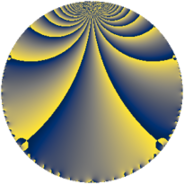# Properties

 Label 8280.2.a.fLevel $8280$ Weight $2$ Character orbit 8280.a Self dual yes Analytic conductor $66.116$ Analytic rank $1$ Dimension $1$ CM no Inner twists $1$

# Related objects

Show commands: Magma / PariGP / SageMath

## Newspace parameters

comment: Compute space of new eigenforms

[N,k,chi] = [8280,2,Mod(1,8280)]

mf = mfinit([N,k,chi],0)

lf = mfeigenbasis(mf)

from sage.modular.dirichlet import DirichletCharacter

H = DirichletGroup(8280, base_ring=CyclotomicField(2))

chi = DirichletCharacter(H, H._module([0, 0, 0, 0, 0]))

N = Newforms(chi, 2, names="a")

//Please install CHIMP (https://github.com/edgarcosta/CHIMP) if you want to run this code

chi := DirichletCharacter("8280.1");

S:= CuspForms(chi, 2);

N := Newforms(S);

 Level: $$N$$ $$=$$ $$8280 = 2^{3} \cdot 3^{2} \cdot 5 \cdot 23$$ Weight: $$k$$ $$=$$ $$2$$ Character orbit: $$[\chi]$$ $$=$$ 8280.a (trivial)

## Newform invariants

comment: select newform

sage: f = N # Warning: the index may be different

gp: f = lf \\ Warning: the index may be different

 Self dual: yes Analytic conductor: $$66.1161328736$$ Analytic rank: $$1$$ Dimension: $$1$$ Coefficient field: $$\mathbb{Q}$$ Coefficient ring: $$\mathbb{Z}$$ Coefficient ring index: $$1$$ Twist minimal: no (minimal twist has level 2760) Fricke sign: $$1$$ Sato-Tate group: $\mathrm{SU}(2)$

## $q$-expansion

comment: q-expansion

sage: f.q_expansion() # note that sage often uses an isomorphic number field

gp: mfcoefs(f, 20)

 $$f(q)$$ $$=$$ $$q - q^{5}+O(q^{10})$$ q - q^5 $$q - q^{5} - 2 q^{11} - 6 q^{17} + 8 q^{19} + q^{23} + q^{25} + 8 q^{29} - 8 q^{31} - 2 q^{37} - 6 q^{41} + 6 q^{43} + 4 q^{47} - 7 q^{49} + 6 q^{53} + 2 q^{55} + 2 q^{61} - 2 q^{67} - 14 q^{71} + 6 q^{73} + 16 q^{79} + 6 q^{85} - 16 q^{89} - 8 q^{95} - 4 q^{97}+O(q^{100})$$ q - q^5 - 2 * q^11 - 6 * q^17 + 8 * q^19 + q^23 + q^25 + 8 * q^29 - 8 * q^31 - 2 * q^37 - 6 * q^41 + 6 * q^43 + 4 * q^47 - 7 * q^49 + 6 * q^53 + 2 * q^55 + 2 * q^61 - 2 * q^67 - 14 * q^71 + 6 * q^73 + 16 * q^79 + 6 * q^85 - 16 * q^89 - 8 * q^95 - 4 * q^97

## Embeddings

For each embedding $$\iota_m$$ of the coefficient field, the values $$\iota_m(a_n)$$ are shown below.

For more information on an embedded modular form you can click on its label.

comment: embeddings in the coefficient field

gp: mfembed(f)

Label $$\iota_m(\nu)$$ $$a_{2}$$ $$a_{3}$$ $$a_{4}$$ $$a_{5}$$ $$a_{6}$$ $$a_{7}$$ $$a_{8}$$ $$a_{9}$$ $$a_{10}$$
1.1
 0
0 0 0 −1.00000 0 0 0 0 0
 $$n$$: e.g. 2-40 or 990-1000 Significant digits: Format: Complex embeddings Normalized embeddings Satake parameters Satake angles

## Atkin-Lehner signs

$$p$$ Sign
$$2$$ $$1$$
$$3$$ $$-1$$
$$5$$ $$1$$
$$23$$ $$-1$$

## Inner twists

This newform does not admit any (nontrivial) inner twists.

## Twists

By twisting character orbit
Char Parity Ord Mult Type Twist Min Dim
1.a even 1 1 trivial 8280.2.a.f 1
3.b odd 2 1 2760.2.a.k 1
12.b even 2 1 5520.2.a.k 1

By twisted newform orbit
Twist Min Dim Char Parity Ord Mult Type
2760.2.a.k 1 3.b odd 2 1
5520.2.a.k 1 12.b even 2 1
8280.2.a.f 1 1.a even 1 1 trivial

## Hecke kernels

This newform subspace can be constructed as the intersection of the kernels of the following linear operators acting on $$S_{2}^{\mathrm{new}}(\Gamma_0(8280))$$:

 $$T_{7}$$ T7 $$T_{11} + 2$$ T11 + 2 $$T_{13}$$ T13 $$T_{17} + 6$$ T17 + 6

## Hecke characteristic polynomials

$p$ $F_p(T)$
$2$ $$T$$
$3$ $$T$$
$5$ $$T + 1$$
$7$ $$T$$
$11$ $$T + 2$$
$13$ $$T$$
$17$ $$T + 6$$
$19$ $$T - 8$$
$23$ $$T - 1$$
$29$ $$T - 8$$
$31$ $$T + 8$$
$37$ $$T + 2$$
$41$ $$T + 6$$
$43$ $$T - 6$$
$47$ $$T - 4$$
$53$ $$T - 6$$
$59$ $$T$$
$61$ $$T - 2$$
$67$ $$T + 2$$
$71$ $$T + 14$$
$73$ $$T - 6$$
$79$ $$T - 16$$
$83$ $$T$$
$89$ $$T + 16$$
$97$ $$T + 4$$